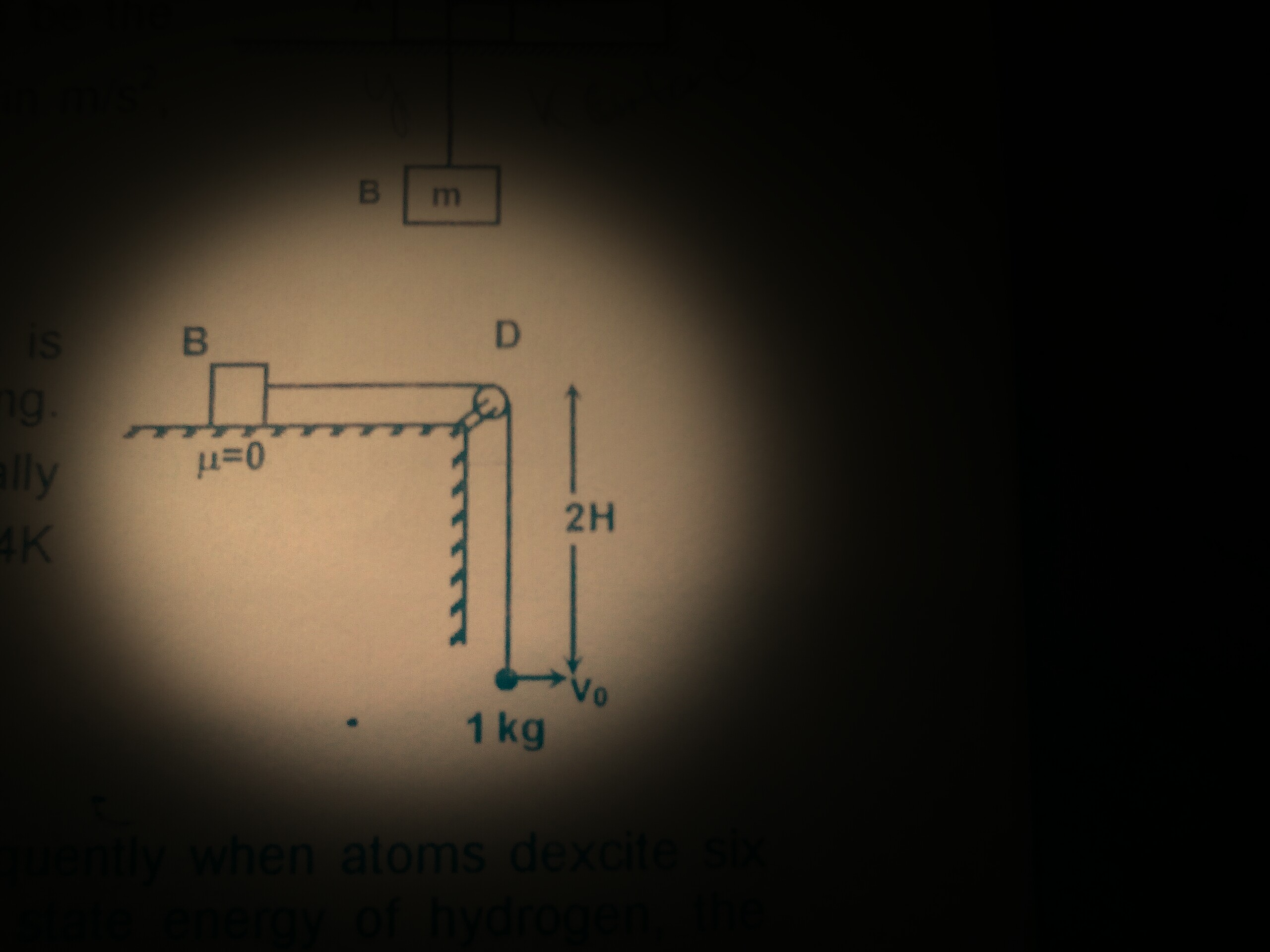# Cool PulleyThe figure above shows a block B of negligible mass connected to a particle of mass $1 \text{ kg}$ with a non-stretchable string. The hanging particle, at rest, is given a velocity $\sqrt{8gH} \text{ m/s}$ horizontally. The initial acceleration of block B is $K \text{ m/s}^2$. Find the value of $K$.

Details:

• Acceleration due to gravity $g=10 \text{ m/s}^2$.
• $H=2 \text{ m}$.
×